# Physics - Classical Mechanics - Rotation with constant angular acceleration[Image 1]

## Introduction

Hey it's a me again @drifter1! Today we continue with Physics and more specifically the branch "Classical Mechanics" to get into Rotations with constant acceleration. So, without further ado, let's get straight into it!

## Constant angular acceleration

In the previous article we described the basic rotational quantities θ, ω and a and how they are related to each other. For particles in rotational (or angular) motion we define:
• θ as the position or angular angle
• ω as the angular velocity
• a as the angular acceleration
These quantities are related to each other as following: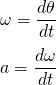The average angular velocity is simply equal to half the sum of the initial and final values: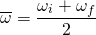Changing the d's with Δ's (and so talking about change of angle over change of time), in the first equation for ω, we can calculate the average angular velocity also with:Solving for θ and setting ti = 0 we get: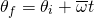Thinking about the constant angular acceleration over some period of time (initial to final) and angular velocity from some initial to some final value, we can write the following integral: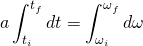Setting the initial time ti to zero again and rearranging we obtain:This equation looks very similar to the linear kinematic equation vf = vi + at.
I guess you can already foresee that the equation for the position/angle will also look quite similar! Doing similar stuff with the equation for angular velocity we can easily find an equation that gives us the final angle after some period of time, when knowing the initial angle and velocity and also the constant angular acceleration of the motion. So, starting off with the equation for angular velocity and substituting what we obtained before we get:Again setting ti to zero and afterwards rearranging we get:An equation very similar to the equation for linear motion: sf = si + uit + 1/2at2.
Solving the equation for angular velocity for time t and substituting it into the equation for angular angle, we can get an equation that's independent of time (I will spare the calculations this time):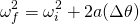Yet another equation similar to liner motion's: vf2 = vi2 + 2a (Δs).

So, by doing all this we ended up with 4 very useful equation that let us calculate:
• Angular displacement from average angular velocity
• Angular velocity from angular acceleration
• Angular displacement from angular velocity and angular acceleration
• Angular velocity from angular displacement and angular acceleration

## Example from Reference_2

Consider a fishing reel that's initially at rest. The fishing line unwinds from the reel at a radius of 4.5cm from it's axis of rotation (suppose that this radius doesn't change as it unwinds). The reel is given a constant angular acceleration of 110 rad/s2 for 2s.
Calculate:
1. The final angular velocity of the reel after 2s
2. How many revolutions the reel makes

### 1.

We know the acceleration a and time t and want to determine ω. For this we can use the "second" equation. Since the initial angular velocity is zero (ωi = 0) the final angular velocity is the only unknown quantity. Therefore we have:### 2.

A revolutions is 1 rev = 2π rad and so to find the number of revolutions we first have to find the θ in radians. We know the values of a and t and that ωi = 0, so we can obtain θ easily using the "third" equation:The number of revolutions is: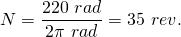As you can see, examples around constant angular acceleration are quite simple, as we just have to apply the 4 kinematic equation that we proved today!

## RESOURCES:

### Images

1.## Final words | Next up

This is actually it for today's post! Next time we will get into Angular Kinetic Energy...

See ya!Keep on drifting!

H2
H3
H4
3 columns
2 columns
1 column